# Need a little help (transformer)

jim hardy
Science Advisor
Gold Member
Dearly Missed
im, the impedance analyzer I'm using is this: http://www.waynekerrtest.com/global/html/products/LCR/6430 6440.htm

It makes a measurement by applying an AC voltage (or current) and measuring (4 terminals) the resulting current (or voltage) with a phase sensitive detector and thereby getting an in phase (real) and out of phase (reactive) value, then calculating things like impedance, admittance, Q, D, etc. I can also make a 4 terminal DC measurement.

That's quite a machine you have there.
From its datasheet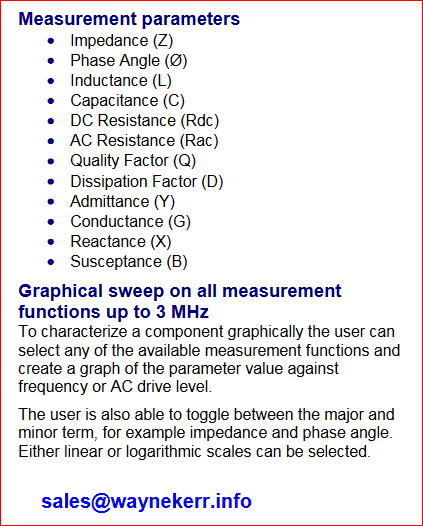Have you looked at a graph of inductance (or impedance) versus frequency?
Over audio range
Air core inductance should be pretty constant but iron core should not, it'll start higher and approach air value as less and less of the iron participates. Remember magnetism proceeds from outside inward and at high frequency it doesn't have time to get beyond skin deep.
At least that's how i explained my results from those photos. My core was an exotic stainless steel used for control rod lifting mechanisms in the reactor.. 400 series stainless makes good solenoid cores because its high resistivity means less eddy currents.

I sure wish we'd had such an instrument when we did our tests back in '92. I neglected to get enough phase measurements to completely characterize our sensor..my bad.
We were investigating temperature sensitivity of the control rod position indicators, basically they measure 60 hz inductance of the 12 foot tall coil as the steel shaft moves up inside it carrying the rod below. It woulda worked a lot better at 3hz, i will always believe. Those photos were an in-situ test from control room . .

As the song goes - "Those were the days my friend! "

------------------------------------------------------------------------------------------------------------------------------------------------------------------------

But i have digressed a long way from the OP's subject. I apologize. To get back to it....
A transformer is an inductor with another coil sharing the region where it makes flux. To understand transformers it is first necessary to understand the humble inductor and that's why i digressed. Sorry if i derailed the thread....

Merlin's voltage readings gives us a number for how much flux couples the secondary coil, but we don't know how much more flux the primary coil made. And we don't know how much flux got cancelled by eddy currents in the core.
I set it up with 0.506A AC 50Hz and measured a primary voltage of 0.96V and secondary voltage of 0.053V
The voltage across the resistance should be 0.86V , which, assuming only an inductive component at 90°, gives a voltage across the inductance of 0.43V.

I think if we knew the phase angles between primary amps and both windings' voltages we'd be better positioned to figure it out.

Anyhow, as electrician said it's going to take a lot of current to make significant flux in OP's device.
And if as N O observed OP's primary is wound half one way and half opposing, well he's built a low ohm non-inductive resistor.

old jim

NascentOxygen
Staff Emeritus
Science Advisor
The bolt was so hot that it heated up the windings and their resistance increased
If the copper coil expanded in diameter then its L would increase. If the wire's gauge expanded, the compression tending to fill in small spacings between turns, I think this would increase inter-turn flux linkage and slightly increase L.

The Electrician
Gold Member
If the wire diameter increases, that effect alone, ignoring compression of the entire coil, will decrease the inductance. There may be other effects not considered.
All that is easy to test. With the bolt at room temperature, I measured the following @50 Hz:
R = .647 ohms, L = 230.1 uH

with bolt removed:
R = .647 ohms, L = 43.017 uH
------------------------------------------------------------
With hot bolt inserted in form for 10 seconds:
R = .783 ohms, L = 294.3 uH

Remove bolt and quickly measure:
R = .748 ohms, L = 42.998 uH

The resistance of the wire slowly decreased back to the pre-heat value of .647 ohms.

The inductance of the coil alone decreased (very little) as a result of heating.

•jim hardy
... I used an ac source of 16 volt....I found that voltage across the primary coil is about 2 volt

I don't know if it's still 'alive' or not, but I think the problem would be about that AC source, which is most likely some small transformer itself, probably capable only up to a few VA output. With such setup the limiting factor would be the resistance of the primary of the source transformer.

Another limiting factor might be the measurement device - cheap multimeters are not really good with measuring low voltage AC (below 1.2 or 0.6V, depending on how cheap the stuff is).

Last edited:
jim hardy
Science Advisor
Gold Member
Dearly Missed
If the wire diameter increases, that effect alone, ignoring compression of the entire coil, will decrease the inductance. There may be other effects not considered.
All that is easy to test. With the bolt at room temperature, I measured the following @50 Hz:
R = .647 ohms, L = 230.1 uH

with bolt removed:
R = .647 ohms, L = 43.017 uH
------------------------------------------------------------
With hot bolt inserted in form for 10 seconds:
R = .783 ohms, L = 294.3 uH

Remove bolt and quickly measure:
R = .748 ohms, L = 42.998 uH

The resistance of the wire slowly decreased back to the pre-heat value of .647 ohms.

The inductance of the coil alone decreased (very little) as a result of heating.

to slide rule accuracy of 3 significant figures :
coil by itself without bolt: 43.0 uH regardless hot or cold
with bolt cold 230 uH
with bolt hot 294 uH

the about six fold increase in inductance by adding the core surprises me, i suspect that for a longer coil the increase would be less dramatic

but clearly temperature affects the iron core's effective permeability
Ur 294/43 = 6.84 hot
Ur 230/43 = 5.35 cold

There's a pretty good derivation here for a long thin solenoid
he starts with Biot Savart, simplifies to a current sheet instead of individual turns
and comes to the familiar equation we all use which as Merlin said gives flux density as function of just mmf amp-turns per unit length,
http://info.ee.surrey.ac.uk/Workshop/advice/coils/air_coils.html

If b >> a (the solenoid is long and thin) then the angles ψ1 and ψ2 will both tend to zero for a point in the middle
B = μ0 Fl teslas Equation ACT (F1 he derives earlier as mmf amps/meter)

At the end of a long coil the flux will be exactly half this value. Only towards the ends does the flux 'leak' through the sides.

In a short coil considerable flux "leaks" out at both ends.

I haven't tried to calculate how much as a function of solenoid's length. I rely on my observations that inserting a core into my 12 foot coil just about doubled its flux. Its length do diameter ratio i'd estimate from memory around thirty-five.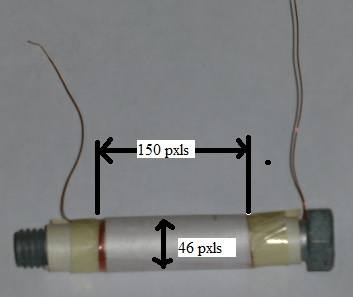length to diameter : 150/46 = 3.2 , if i understood .

Ohhh the things they never mentioned in undergrad class ....
http://www.g3ynh.info/zdocs/magnetics/Solenoids.pdf page 32 of 97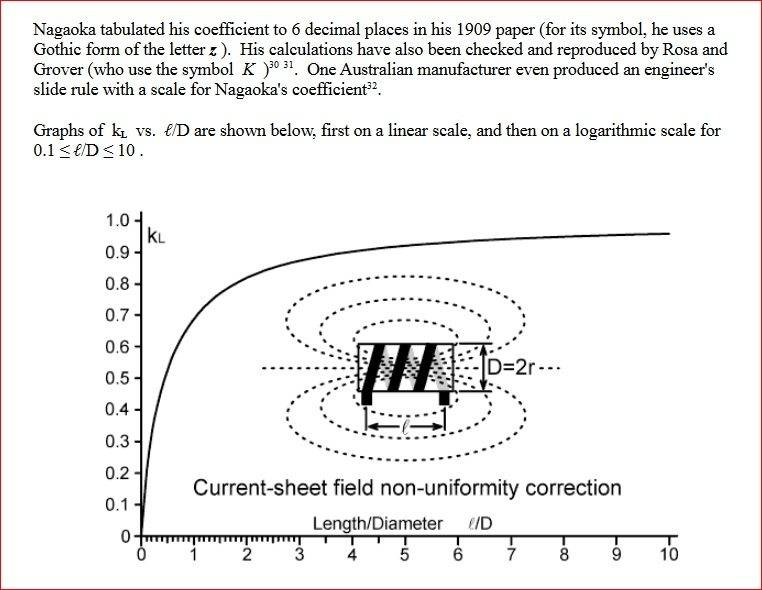Last edited:
The Electrician
Gold Member
I misspoke in post #53; the frequency of the measurement was 1000 Hz, not 50 Hz. Refer back to post #43 where the 50 Hz inductance with the bolt in place was 608 uH. At 50 Hz, the measured inductance is increased about 14 times by the insertion of the bolt.

You asked about the inductance as a function of frequency. Here's a sweep of the inductance and AC resistance measured at the primary (the 100 turn winding). The frequency is swept from 10 Hz to 1 MHz. The scale for the inductance curve (green) varies from 1 uH at the bottom of the image to 1000 uH at the top. The scale for AC resistance (real part of the impedance) curve (yellow) varies from .1 ohm at the bottom to 100 ohms at the top. There are two markers, A and B. The values of inductance and resistance at the marker frequencies (50 Hz and 10 kHz) can be read at the top right of the image. This sweep is for the coil without the bolt inserted ("air" core, in other words):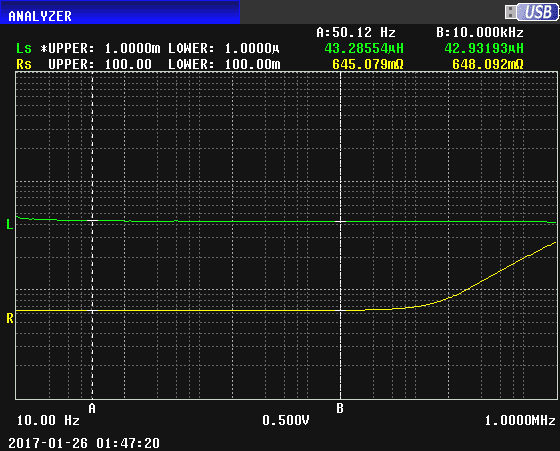Notice how the inductance is constant with frequency and the resistance is constant until it begins to rise in the neighborhood of 50 kHz due to skin and proximity effect.

Now with the bolt inserted: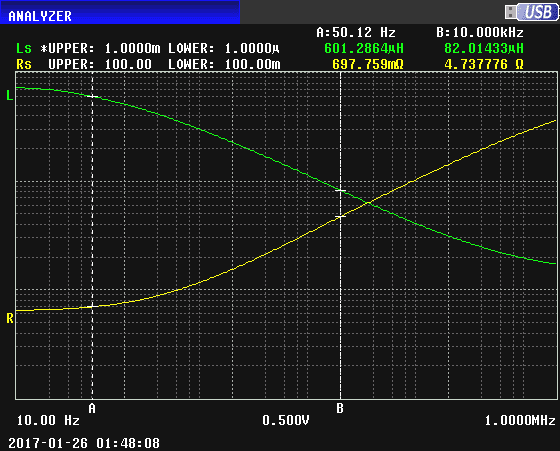We see inductance decreasing drastically with increasing frequency, and resistance increasing.

Here are both sweep results superimposed: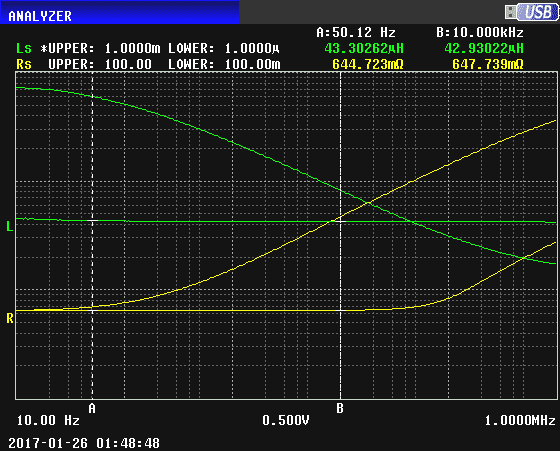Notice how the inductance with the bolt inserted actually becomes less than the "air" core value as the frequency increases above about 45 kHz. At such high frequencies, the bolt just looks a shorted turn with very little penetration of flux into the bolt.

•NascentOxygen and jim hardy
The Electrician
Gold Member
Another limiting factor might be the measurement device - cheap multimeters are not really good with measuring low voltage AC (below 1.2 or 0.6V, depending on how cheap the stuff is).

No doubt. Have a look at the values I measured in post #45. The OP definitely needs to use a DVM with a millivolt scale.

•jim hardy